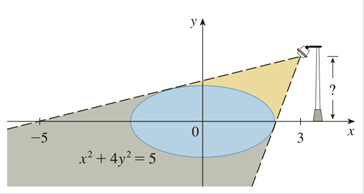Chapter 2.6, Problem 62E

Chapter
Section
Textbook Problem

# The figure shows a lamp located three units to the right of the y-axis and a shadow created by the elliptical region x 2 + 4 y 2 ≤ 5 . If the point ( − 5 ,   0 ) is on the edge of the shadow, how far above the x-axis is the lamp located?To determine

To Find:

Distance between lamp and x-axis

Solution:2 units

Explanation

1) Formula:

i. Sum or difference rule

ddxfx ±gx=ddx fx± ddxgx ;

ii. Derivative of constant

ddxc=0,

iii. Product rule

ddxfx*gx=fxddxgx+gxddx fx

iv. Power rule

ddxxn=n xn-1

2) Calculation:

We have the equation of ellipse x2+4y2=5.

Take derivative on both sides of the equation with respect to y,

We get,

ddx(x2+4y2)=ddx(5)

Apply Sum and Difference rule of differentiation

ddx5=0 by using constant rule of differentiation So we have

2x+42ydydx=0

Solving for dydx

m=dydx=-2x8y=-x4y This is the slope of tangent to the ellipse at point (x,y)

### Still sussing out bartleby?

Check out a sample textbook solution.

See a sample solution

#### The Solution to Your Study Problems

Bartleby provides explanations to thousands of textbook problems written by our experts, many with advanced degrees!

Get Started

#### Find more solutions based on key concepts